# 机器学习算法入门之(一) 梯度下降法实现线性回归

## 1. 背景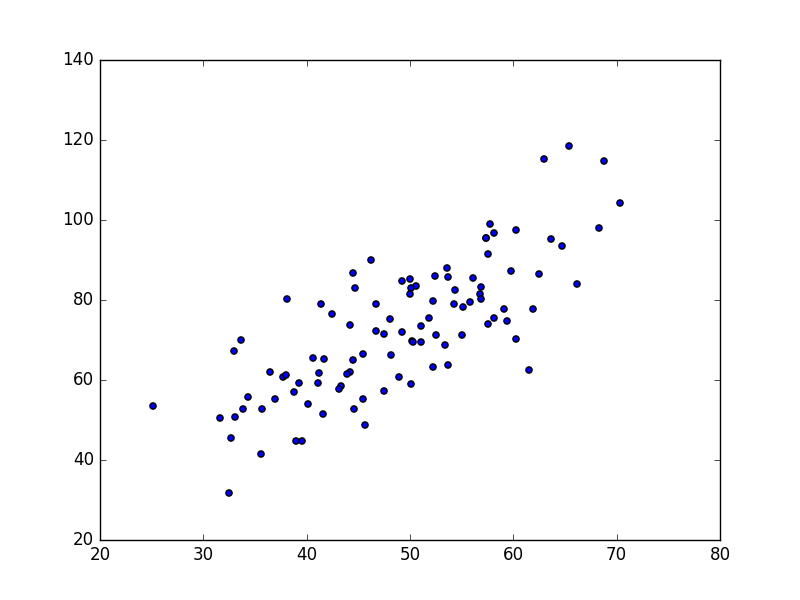Error(b,m)=1N1N((b+mxi)yi)2

# y = b + mx
def compute_error_for_line_given_points(b, m, points):
totalError = sum((((b + m * point) - point) ** 2 for point in points))
return totalError / float(len(points))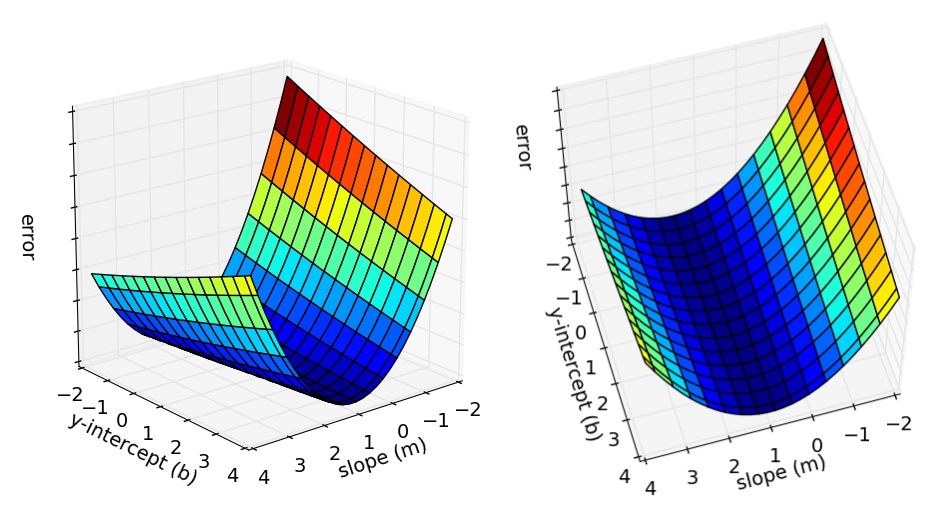## 2. 多元线性回归模型

hθ(x)=θ0+θ1x1+...+θnxn=θTxx0=1
J(θ)=12i=1m(hθ(x(i))y(i))2mm

### 2.1 误差函数的进一步思考

y(i)=θTx(i)+ε(i)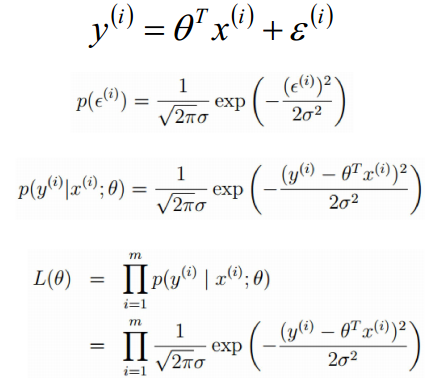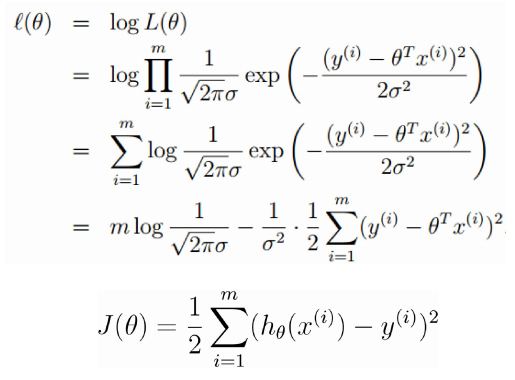## 3 最小二乘法求误差函数最优解

J(θ)=12i=1m(hθ(x(i))y(i))2=12(XθY)T(XθY)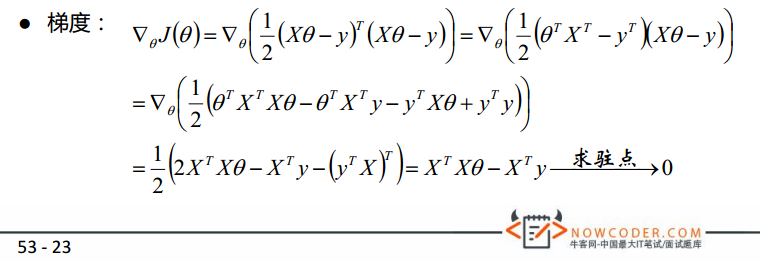### 3.1 python实现最小二乘法

# 通过最小二乘法直接得到最优系数，返回计算出来的系数b, m
def least_square_regress(points):
x_mat = np.mat(np.array([np.ones([len(points)]), points[:, 0]]).T)  # 转为100行2列的矩阵，2列其实只有一个feature，其中x0恒为1
y_mat = points[:, 1].reshape(len(points), 1)  # 转为100行1列的矩阵
xT_x = x_mat.T * x_mat
if np.linalg.det(xT_x) == 0.0:
print('this matrix is singular,cannot inverse')  # 奇异矩阵，不存在逆矩阵
return
coefficient_mat = xT_x.I * (x_mat.T * y_mat)
return coefficient_mat[0, 0], coefficient_mat[1, 0] # 即系数b和m

b = 7.99102098227, m = 1.32243102276, error = 110.257383466, 相关系数 = 0.773728499888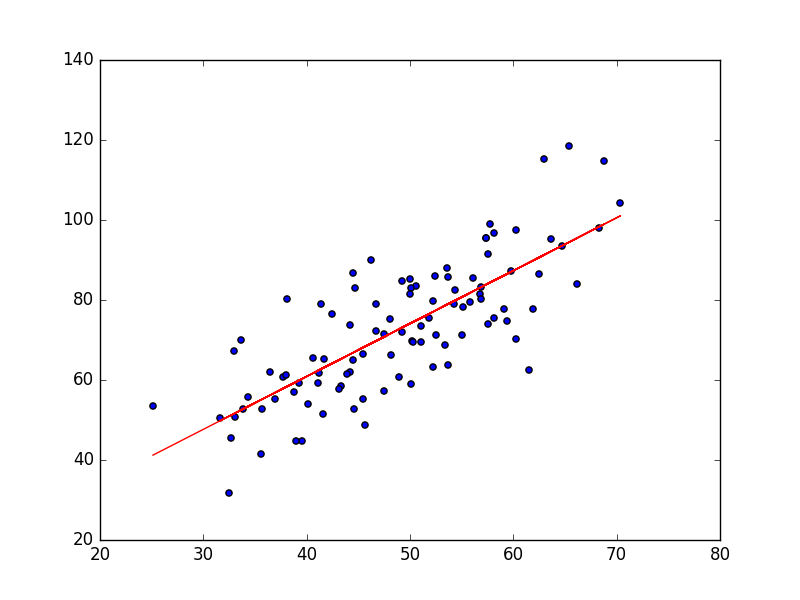## 4. 梯度下降法求误差函数最优解

### 4.1. 梯度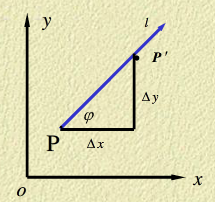fl=limρ0f(x+Δx,y+Δy)f(x,y)ρρ=(Δx)2+(Δy)2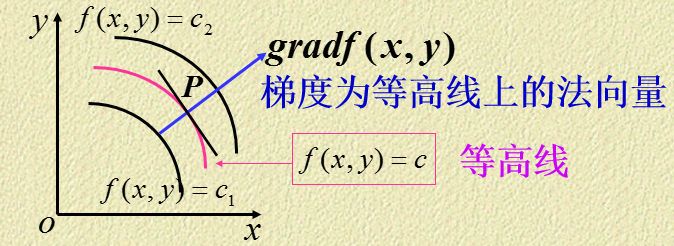### 4.2 梯度方向计算

Error(b,m)m=i=1Nxi((b+mxi)yi)
Error(b,m)b=i=1N((b+mxi)yi)x01

θjJ(θ)=θj12i=1m(hθ(x(i))y(i))2=i=1m(hθ(x(i))y(i))x(i)j

### 4.3 批量梯度下降法

θj=θjαJ(θ)θj=θjαi=1m(hθ(x(i))y(i))x(i)j

def step_gradient(b_current, m_current, points, learningRate):
N = float(len(points))
for i in range(0, len(points)):
x = points[i, 0]
y = points[i, 1]
m_gradient += (2 / N) * x * ((b_current + m_current * x) - y)
b_gradient += (2 / N) * ((b_current + m_current * x) - y)
new_b = b_current - (learningRate * b_gradient)  # 沿梯度负方向
new_m = m_current - (learningRate * m_gradient)  # 沿梯度负方向
return [new_b, new_m]

# this is the instance used by the matplotlib classes
rcParams = rc_params()

# fix a bug by ZZR
rcParams['animation.convert_path'] = 'C:\Program Files\ImageMagick-6.9.2-Q16\convert.exe'

learningRate=0.0001，迭代100轮的结果如下图：

After {100} iterations b = 0.0350749705923, m = 1.47880271753, error = 112.647056643, 相关系数 = 0.773728499888
After {1000} iterations b = 0.0889365199374, m = 1.47774408519, error = 112.614810116, 相关系数 = 0.773728499888
After {1w} iterations b = 0.607898599705, m = 1.46754404363, error = 112.315334271, 相关系数 = 0.773728499888
After {10w} iterations b = 4.24798444022, m = 1.39599926553, error = 110.786319297, 相关系数 = 0.773728499888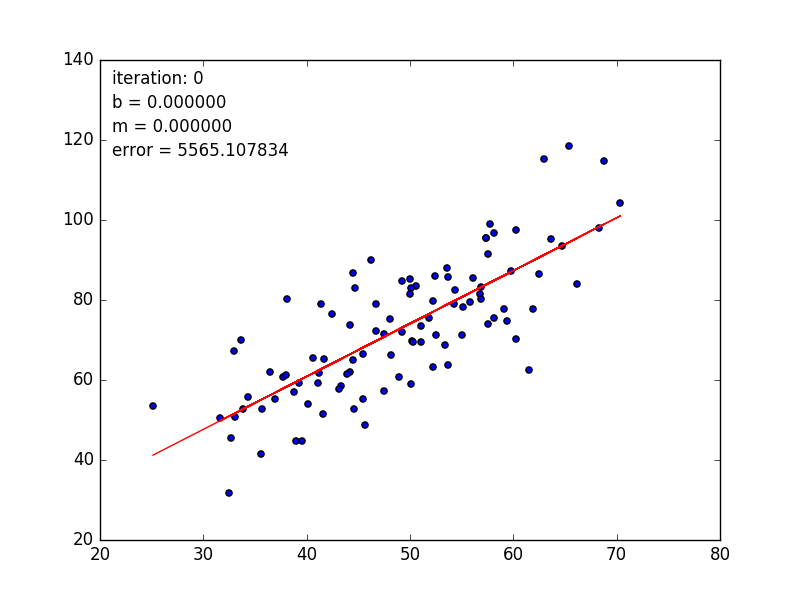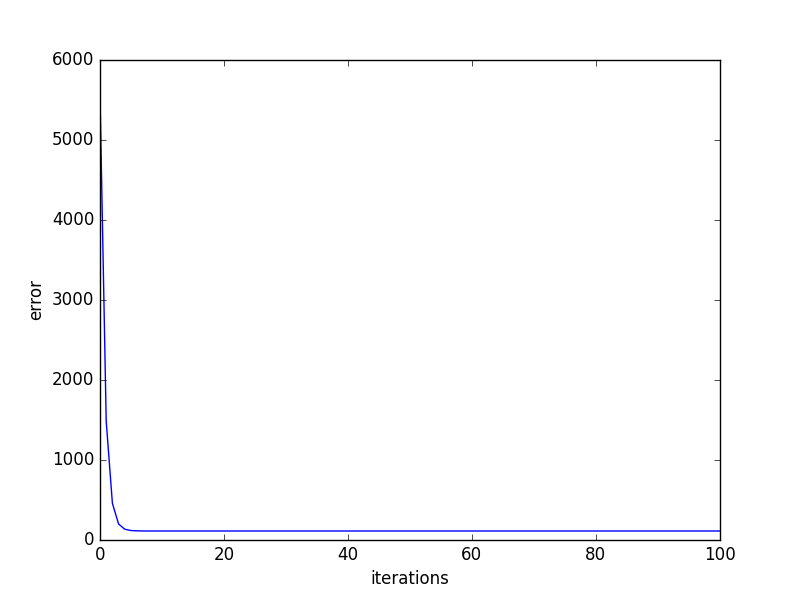### 4.4 随机梯度下降法

θj=θjα(hθ(x(i))y(i))x(i)j

## 参考文献